## Example Questions

2 Next →

### Example Question #224 : Geometry

Find the area of a square whose side length is 5.Explanation:

To solve, simply use the formula for the area of a square. Thus,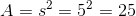### Example Question #225 : Geometry

A rhombus that is also a rectangle has a side length of. What is its area?

Cannot be determined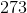Explanation:

Remember that a rhombus that is also a rectangle is a square. Knowing that, the calculation is easy: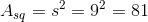### Example Question #226 : Geometry

One of the sides of a square on the coordinate plane has an endpoint at the point with coordinates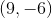; it has the origin as its other endpoint. What is the area of this square?None of the other responses gives a correct answer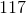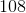Explanation:

The length of a segment with endpoints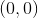andcan be found using the distance formula with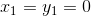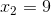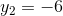: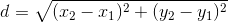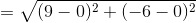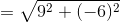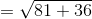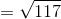This is the length of one side of the square, so the area is the square of this, or 117.

### Example Question #227 : Geometry

A circle is inscribed by a square whose sides are five units long such that the sides of the square barely touch the circle. What is the area inside the square that is not occupied by the circle?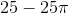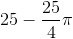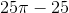Explanation:

We want to find the area inside the square that is not taken up by the circle. With that in mind, we can say that the area we're looking for can be expressed by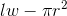. In other words we are subtracting the area of the circle from the area of the square. This will give us the desired area.

Now, substitute our known values in for the variables to find the desired area: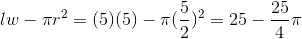### Example Question #228 : Geometry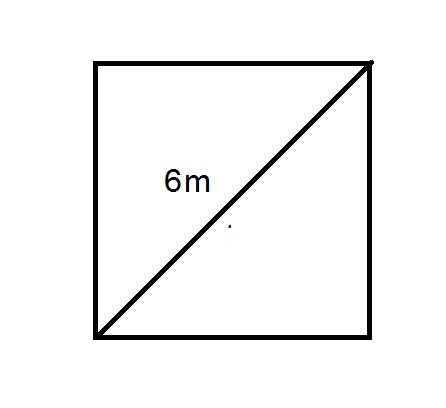Give the area of the provided square in square centimeters.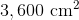None of these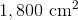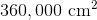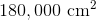Explanation:

The diagonal shown has length 6 meters; to convert to centimeters, multiply by 100: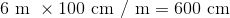The easiest way to find the area of the square given the length of a diagonal is to note that since a square is a rhombus, its area is equal to half the product of the lengths of its diagonals. Since one diagonal has length 600 centimeters, so does the other, and the area of the square is therefore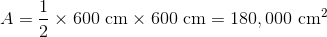### Example Question #229 : Geometry

A square has the following perimeter: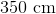Express its area in square meters.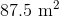None of these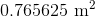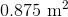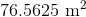Explanation:

One meter comprises 100 centimeters, so divide the perimeter of the sides in centimeters by 100 to obtain the length in meters. This is the same as moving the decimal point left two spaces: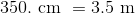The perimeter of a square is equal to the sum of the lengths of its four equally long sides, so the length of one side is one fourth of - or, in decimal form, 0.25 times - its perimeter. For this square, this is: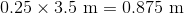The area of a square is equal to the square of the length of one side, so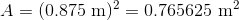### Example Question #11 : How To Find The Area Of A Square

Give the area of a square with the following perimeter: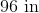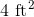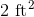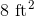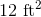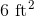Explanation:

The perimeter of a square is equal to the sum of the lengths of its four equally long sides, so the length of one side is one fourth of its perimeter. For this square, this is: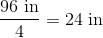12 inches are equal to one foot, so divide by 12 to convert to feet: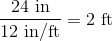The area of a square is equal to the square of the length of one side, so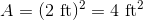### Example Question #83 : Quadrilaterals

If the perimeter of a square is equal to twice its area, what is the length of one of its sides?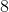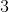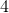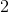Explanation:

Area of a square in terms of each of its sides:

Area = S x S

Perimeter of a square:

Perimeter = 4S

So if 'the perimeter of a square is equal to twice its area':

2 x Area = Perimeter

2 x [S x S] = [4S]; divide by 2:

S x S = 2S; divide by S:

S = 2

2 Next →